# Numpy square root explained

This tutorial will cover the NumPy square root function, which is also called numpy.sqrt or np.sqrt.

This is a fairly easy NumPy function to understand and use, but for the sake of helping true beginners, this tutorial will break everything down. I’ll quickly review NumPy, I’ll explain the syntax, and I’ll show you some examples.

That being said, if you’re in a hurry, you can click on one of the links to the following sections and get what you’re looking for.

Contents:

If you’re a real beginner or you have some time on your hands, I recommend that you read the whole tutorial. Everything will make more sense that way.

But if you’re in a hurry, click on one of the links in the Contents list, and it will take you directly to the appropriate section of the tutorial.

Ok … before we get into the Numpy square root function itself, let’s start with a quick review of NumPy.

## A quick review of NumPy

The NumPy square root function is part of the NumPy module.

So what is NumPy?

NumPy is a toolkit for performing computing with numeric data in Python.

Base Python itself has many functions for working with numeric data, but Numpy has been carefully designed to work with large arrays of numbers.

What is a Numpy array?

A Numpy array is just a special data structure for storing numbers. You can think of a Numpy array as a grid of numbers.

In simple cases, these arrays can be 1-dimensional:In more complicated cases, Numpy arrays can be 2-dimensional:Numpy also supports 3D arrays, and higher dimensional arrays.

So what makes NumPy important is that it provides a variety of tools for creating arrays of numbers, manipulating those arrays, and performing mathematical computations on those arrays.

One tool for performing calculations on a NumPy array is the NumPy square root function.

## A quick introduction to NumPy square root

So what does numpy.sqrt do?

#### numpy.sqrt calculates a square root in Python

To put it simply, the NumPy square root function calculates the square root of input values.

So if you give it an input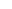, numpy.sqrt() will calculate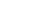: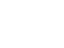#### numpy.sqrt also works on arrays

Critically though, the Numpy square root function also works on Numpy arrays.

So if you give it a Numpy array as an input, Numpy square root will calculate the square root of every value in the array.

I’ll show you examples of both cases in the examples section, but first let’s take closer look at the syntax.

## The syntax of np.sqrt

The syntax of NumPy square root is extremely simple. It’s probably one of the simplest functions in the NumPy module.

To call the function, you just call it by name (although exactly how you call it depends on how you’ve imported NumPy).

##### An important note about importing NumPy

When you initially import NumPy into your environment, you can simply do this with the code import numpy. This will enable you to call NumPy functions with the prefix “numpy.” followed by the name of the function.

However, it is very common to give NumPy a “nickname” when it’s imported. It’s very common to import NumPy with the code import numpy as np. This essentially gives NumPy the alias np in your code, which enables you to use “np.” instead of “numpy.” when you call functions.

This is important, because how you import numpy will determine how you call it in your code.

Since it’s common to import NumPy with the code import numpy as np, we’ll follow that convention in our syntax.

Just be aware though that np.sqrt() and numpy.sqrt() are essentially interchangeable, as long as you’ve imported NumPy the correct way, respectively.

### np.sqrt syntax

Assuming that we’ve imported NumPy with the code import numpy as np, we call call the NumPy square root function with the code np.sqrt().Then inside of the function, there is a parameter that enables you to specify the input of the function.

Let’s quickly discuss this.

### The parameters of numpy.sqrt

The NumPy square root function actually has two parameters, but we’re really only going to talk about one of them.

Parameters:

• x
• out

Let me quickly explain each of these separately.

###### x (required)

The x parameter enables you to specify the input to the np.sqrt function.

Technically, the x parameter enables you to specify the radicand. The radicand is the value under the radical when you compute the square root.

Keep in mind that the function is somewhat flexible in what types of inputs it will accept as arguments to the x parameter. You can provide a single number, but you can also provide a NumPy array or any array-like input. The array-like inputs that will work are things like Python lists and tuples.

If you provide a NumPy array or array-like input, numpy.sqrt will compute the square root of each value in the array.

I’ll show you examples of all of these cases in the examples section.

Note that you need to provide an argument to the x parameter, meaning that you need to provide some sort of input to the function … an integer, an array, a list. You need to provide something as an input.

###### out

The out parameter enables you to specify an array where the output will be stored.

This parameter is not commonly used by beginners, so we’re not really going to work with it in this tutorial.

Ok, now that I’ve explained the syntax and the parameters, let’s take a look at some examples.

## Examples of how to use NumPy square root

As I mentioned earlier, Numpy square root is very easy to use.

Having said that, I’m still going to start with the simplest possible example, and then increase the complexity as we move on to other examples.

Examples:

#### Run this code first

One quick note.

As I mentioned earlier, you’ll need to import NumPy into your environment in order for the code to work properly.

You can do that by running this code:

import numpy as np


This imports the NumPy module with the alias “np“. As I mentioned previously, this is a common convention among NumPy users. Ultimately, it enables us to refer to NumPy as np in our code, so the prefix “np.” will be in front of the function name when we call a function.

### Use np.sqrt on a single number

First, let’s start simple.

Here, we’re going to apply the NumPy square root function to a single numeric input.

np.sqrt(9)


OUT:

3.0


So what happened here?

We called the function with the code np.sqrt(9). This is essentially the same as np.sqrt(x = 9), but we can chose not to explicitly type the x =. The code works the same way in either case.

And what did it compute?

This is very simple.

Here, np.sqrt computed the square root: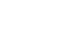As I’m sure you know,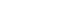, so it gave us the output of 3.0.

### Use np.sqrt with a 1-dimensional array

Next, let’s use the function on a 1-dimensional “array” of numbers.

Actually, for the sake of clarity, I’m going to show you the example with a Python list.

Remember: numpy.sqrt will operate on any array-like structure.

I think that Python lists are a little easier to understand, because they make the syntax easier to understand. For the sake of completeness though, I’ll re-do this example later with a proper NumPy array.

Ok, let’s run the code. Here, we’re going to apply the np.sqrt function to the Python list [0,1,2,3,4].

np.sqrt([0,1,2,3,4])


Which produces the following output:

array([0. , 1. , 1.41421356 , 1.73205081 , 2. ])


So what happened here?

This is really simple.

Remember: when we apply np.sqrt to a list, it computes the square root for every element of the list.So it takes the input values [0,1,2,3,4], computes the square root for each value, and produces the output in the form of a NumPy array.

That’s it!

##### Redo example with a numpy array

Very quickly, let’s redo this example with a proper NumPy array as the input.

So, instead of using the list [0,1,2,3,4], we’re going to use a NumPy array that contains those values instead.

Let’s first create the array:

np_array_1d = np.arange(5)


If you print this out, you’ll see the contents:

print(np_array_1d)


OUT:

[0 1 2 3 4]


So np_array_1d contains the same values as the list that we worked with earlier, but it’s in the form of a NumPy array. Note that you can check the object type with the code type(np_array_1d) … which will show you that it’s a numpy.ndarray.

Ok. So we now have our NumPy array, np_array_1d.

Now, let’s use that as the input to np.sqrt():

np.sqrt(np_array_1d)


Which produces the output:

array([0. , 1. , 1.41421356 , 1.73205081 , 2. ])


Notice that this is the same output that we got for np.sqrt([0,1,2,3,4]).

The point that I’m trying to make here is that np.sqrt will operate on either a list or a NumPy array … in fact, it will work on any array-like object.

### Using np.sqrt with a 2-dimensional array

Next, we’re going to use np.sqrt on a 2-dimensional array.

First, let’s just create the array.

np_array_2d = np.arange(10).reshape([2,5])


This new array, np_array_2d, is a simple 2-dimensional array. It contains the numbers from 0 to 9, arranged in an array with 2 rows and 5 columns. We’re creating this array with the np.arange function, in conjunction with the NumPy reshape method.

You could print it out with print(np_array_2d), but visually, it looks like this:Now, let’s use this array as the input to np.sqrt:

np.sqrt(np_array_2d)


Which produces the following output:

array([[0.        , 1.        , 1.41421356, 1.73205081, 2.        ],
[2.23606798, 2.44948974, 2.64575131, 2.82842712, 3.        ]])


This is really easy to understand, if you’ve been paying attention to the previous examples.

When we use a 2D NumPy array as the input, the np.sqrt function simply calculates the square root of every element of the array.The output of the function is simply an array of those calculated square roots, arranged in exactly the same shape as the input array. So if the input array has 2 rows and 5 columns … then the output array will have 2 rows and 5 columns.

#### Note: all of this extends to multi dimensional arrays

So far, I’ve shown you examples of how the NumPy square root function operates on scalars, 1D arrays, and 2D arrays.

But keep in mind that it will also work on arrays of different sizes and shapes.

So you can apply this function to 3D arrays or higher-dimensional arrays and it works in essentially the same way: it computes the square root of each element.

## NumPy square root FAQ

Let’s quickly take a look at one commonly asked question about NumPy square root.

### Can I calculate the square root of -1 with numpy.sqrt?

Not exactly.

Taking the square root of -1 with np.sqrt causes an warning message:

np.sqrt(-1)

RuntimeWarning: invalid value encountered in sqrt
Out: nan


Essentially, NumPy is saying that the input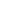is invalid. The code still runs, but the output is “nan” … meaning “not a number.”

##### np sqrt supports complex numbers

Having said that, NumPy supports complex numbers, and the np sqrt function does operate on complex numbers.

np.sqrt(-1+0j)


OUT:

1j


Explaining this example is a little complex (heh heh), and it’s outside the scope of this tutorial.

Ultimately, you need to know that NumPy sqrt does not natively operate on negative numbers, and to get it to do so, you’ll need to use complex numbers.

Do you have other questions about NumPy square root?

## Other Python data science tutorials

If you’re interested in data science in Python, you’ll have a lot to learn … a lot more than just the NumPy square root function.

We have plenty of free tutorials here that you can check out.

NumPy tutorials:

Those are just a few of our NumPy tutorials.

We also have tutorials about Pandas and matplotlib.

Ultimately though, if you want to regularly get our FREE tutorials, you should sign up for our email list.

Members of our email list are notified immediately when we publish new data science tutorials … we basically send the tutorials to your inbox.

If you sign up, you’ll get free tutorials on a variety of data science topics:

• NumPy
• Pandas
• Base Python
• Scikit learn
• Machine learning
• Deep learning
• data science in Python
• data science in R
• … and more.